Next: Parallel Boundaries Up: Description of Three Dimensional Previous: Boundary Curvature

# Boundary Length

Given the three dimensional data, the length of boundary segments can be estimated directly. If the segment is straight, then its length is just the distance between its endpoints. Otherwise, given the groupings described in the previous section, the calculated curvature and the geometric definitions, the length of a curved segment is given as follows (referring to Figure 6.2):

 Let: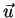be the unit vector in the directionbe the center of the arc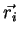be the unit vector from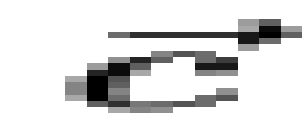to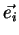Then: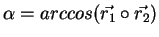is the angle subtended by the arc, and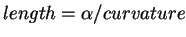The boundary length estimates for some of the complete boundaries in the test image (segment labels from Figure 6.3) are shown in Table 6.2.

Table 6.2: Boundary Length Estimates
REGION SEGMENTS ESTIMATED LENGTH TRUE LENGTH % ERROR
26 1 31.9 25.2 26
26 2 37.0 25.2 47
8 3,4,5 51.1 50.0 2
8 6 27.3 28.2 3
8 7,8,9,10 46.1 50.0 8
9 11,12 28.0 27.2 3
9 13 30.1 34.5 12
9 14,15,16 25.3 27.2 7
9 17,18,19,20 32.7 45.5 28

The average error for boundary length is about 20%, but there are larger errors. On the whole, the estimates are generally acceptable, though not accurate. The poor estimates for segments 1 and 2 result from data errors.Next: Parallel Boundaries Up: Description of Three Dimensional Previous: Boundary Curvature
Bob Fisher 2004-02-26